# Errata

This page contains information about errata for Feedback Systems. The errata listed here include known and corrected errors since the first printing of the book; clicking on the link will take you to a page with additional details. Typos and other relatively minor corrections are listed here but do not have separate pages with additional details (they also do not appear on individual chapter pages).

Conventions:

• Each line of text and each line of a displayed equation are counted as a line. Negative line numbers mean number of lines from the bottom of the page. First line on page = 1, last line on page = -1.
• Entries in boldface indicate errors which might change significantly the interpretation of the text.
• The version refers to the release number in which the error was found. Errors are corrected in all subsequent releases of the text.
• The status of the errata uses the following terms:
• Submitted - erratum has been submitted, but not yet processed
• Open - erratum has been received, but not yet corrected
• Pending - erratum has been corrected, but fix is not yet posted
• Closed - erratum has been corrected and revision is posted
• Rejected - erratum was rejected (see page for information on why)

## Second Edition

### Preprint

PageLineDescriptionVersionStatusDate
1-237In the air-fuel example in Section 1.8, missing reference to Figure 1.16bVersion 3.0hClosed28 September 2016
1-2325In Section 1.8, breaking should be brakingVersion 3.0hClosed28 September 2016
1-237In Section 1.7, there is a typo/missing word near the end of the sectionVersion 3.0hClosed28 September 2016
1-258In Section 1.8 text on paper mills, "production unit" should be "production units"Version 3.0hClosed28 September 2016
1-258In the paper mill description in Section 1.8, "production unit" should be "production units"Version 3.0hClosed28 September 2016
10-15In Section 10.3, the caption for Figure 10.11 seems to have a typoVersion 3.0hClosed13 November 2016
10-18In Section 10.4, Figure 10.14(b) seems to missing the curve for reverse driving phaseVersion 3.0hClosed13 November 2016
10-18In Section 10.4, the Figure 10.14 caption has a grammatical errorVersion 3.0hClosed13 November 2016
10-2-8In Section 10.1, there is a typo in the oscillation condition equationVersion 3.0hClosed13 November 2016
10-2-8In Equation 10.1, there's a typo 0 instead of ')'Version 3.0hDuplicate14 November 2016
10-3-7In Section 10.1, the word "circuit" is misspelled.Version 3.0hClosed13 November 2016
10-7In Section 10.2, part of Figure 10.5 seems to be missingVersion 3.0hClosed13 November 2016
11-128Ziegler-Nichols tuning rules don't quite match the original gains from the Ziegler-Nichols paperVersion 3.0jClosed31 August 2019
11-1322, 23Original Ziegler-Nichols gains listed after improved tuning rules are incorrectVersion 3.0jClosed31 August 2019
11-1322, 23Updated tuning rules are incorrect in equation (11.10b)Version 3.0jClosed31 August 2019
11-16In Section 11.4, the Figure 11.10 caption has a typoVersion 3.0hClosed13 November 2016
11-519In Section 11.1, the word "frequencies" is duplicatedVersion 3.0hClosed13 November 2016
12-10-1In Section 12.2, there is a typo in the equation for P^+(s)Version 3.0hClosed19 November 2016
12-101In Section 12.2, there is a singular vs. plural error when talking about the control signalVersion 3.0hClosed19 November 2016
12-112In Section 12.2, there is a typo in the equation for the approximate inverse of P(s)Version 3.0hClosed19 November 2016
12-11-4In Section 12.2, the word "inherently" is misspelledVersion 3.0hClosed19 November 2016
12-11-4In Section 12.2, the sentence about sensitivity to model uncertainty has a grammatical issueVersion 3.0hClosed19 November 2016
12-14-5In Section 12.3, there is a missing spaceVersion 3.0hClosed19 November 2016
12-1611In Section 12.4, the word "limitations" is misspelledVersion 3.0hClosed19 November 2016
12-28In Section 12.6, there is a missing comma in the caption of Figure 12.17Version 3.0hClosed22 November 2016
12-3-14In Section 12.1, the additional transfer functions for the F != 1 case seem to be incorrectVersion 3.0hClosed19 November 2016
12-3018In Section 12.6, a sentence in Theorem 12.2 is grammatically incorrectVersion 3.0hClosed22 November 2016
12-3511In Section 12.7, there's a grammatical error where it talks about the closed loop system H iVersion 3.0hClosed22 November 2016
12-354In Section 12.7, there's a typo in the discussion of inner/outer loop designVersion 3.0hClosed22 November 2016
12-4-5In Section 12.1, the vector w corresponding to Fig. 12.1 seems to be incorrectVersion 3.0hClosed19 November 2016
12-5In Section 12.2, the feedforward command u fv is mislabeled in Figure 12.3Version 3.0hClosed19 November 2016
12-5-3In Section 12.2, the word "desired" is misspelledVersion 3.0hClosed19 November 2016
12-617In Section 12.2, the condition for Gyv being small seems to be misstatedVersion 3.0hClosed19 November 2016
13-1510In Section 13.3, the word "response" is duplicatedVersion 3.0hClosed22 November 2016
13-6-11In Section 13.2, the word "when" is incorrectly capitalizedVersion 3.0hClosed22 November 2016
13-78In Section 13.2, there's a grammatical issue in the discussion of system stabilityVersion 3.0hClosed22 November 2016
13-79In Section 13.2, the word "transfer" is misspelledVersion 3.0hClosed22 November 2016
13-79In Section 13.2, the word "point" is duplicatedVersion 3.0hClosed22 November 2016
13-8-9In Example 13.6 (Vinnicombe metric), the formula for conjugate point is missing a minus signVersion 3.0jClosed19 August 2019
2-1119In discussion on PI control in Section 2.2, incorrect formula for G yv when \omega is smallVersion 3.0hClosed1 October 2016
2-237In Section 2.1, there is a typo in the discussion of solving for an exponential inputVersion 3.0hClosed30 September 2016
2-49In Section 2.1, referred is misspelledVersion 3.0hClosed30 September 2016
2-55In Example 6.8, signalsith should be signals withVersion 3.0hClosed12 October 2016
2-7-12Equation (2.11) has the wrong formula for the transfer functionVersion 3.0hDuplicate1 October 2016
2-79Equation 2.11 is not equal to the preceding expression from which it cameVersion 3.0hClosed4 October 2016
2-812In Section 2.2, one too many parentheses in an expression for y(t)Version 3.0hClosed4 October 2016
2-815Display equation above equation (2.12) has extra set of parenthesesVersion 3.0hClosed4 October 2016
2-913In discussion of proportional control in Section 2.2, missing v 0 in displayed equationVersion 3.0hClosed1 October 2016
3-1033In Section 3.1, there is a typo for pluralityVersion 3.0hClosed4 October 2016
3-10-8In discussion on algebraic loops in Section 3.2, there is an extra "be"Version 3.0hClosed4 October 2016
... further results

## First Edition

### Third printing

Page Line Erratum Version
10   -1   In the "Instrumentation" subsection, "axon of the giant squid" should be "giant axon of the squid" 2.10c
37   4   In Example 2.1, the state space equations for the balance system are missing a divisor of J_t in one of the terms 2.10c
38   20, 21   In equation (2.12),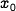should be x (twice) 2.10e
40   2   In Figure 2.7, the coefficients are for an update period of one day 2.10c
42   2   In Figure 2.9, "solid" and "dashed" should be swapped 2.10e
43   12   In displayed equation for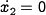, leading minus sign is missing from rightmost expression 2.10d
47   -6   In Example 2.5, expression for the step response is missing some factors 2.10d
49   19   In the normalized state space model for the spring-mass system, the derivative should be with respect to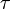2.11b
49   -10   In Example 2.7, reference to the previous example should be Example 2.1, not Section 2.1 2.11b
49   -8 to -4   In Example 2.7, q should be replaced by p (three places) and theta (one place) 2.10d
61   -4   In Exercise 2.3, disrete should be discrete 2.10e
62   -10   In Exercise 2.4, a = 0.25 should be a = 0.75 2.10e
84   11   In the online version of the text, there is a formatting error in equation (3.23) 2.10c
86   -1   In equation (3.26), q_0 is not defined 2.10c
100   -6   In Example 4.4, second simplifying assumption should be l/J_t = 1 (remove extra factor of m) 2.10d
105   -3   On right hand side of displayed equation, x should be x_j 2.10c
107   -7   At the end of Example 4.6, the roots should have negative real part instead of being positive 2.10d
110   -5   Near end of Example 4.11, denominator of f'(z_2e) term should be squared 2.10d
113   -14   In Example 4.10, \dot V = 0 should be treated as negative semidefinite, not positive 2.10d
116   3,6   In Example 4.11, Lyapunov equation entries for f'_1 and f'_2 are switched (solution is correct) 2.10d
121   10   In Example 4.13, inequality should be for x_1 instead of x_2 2.10c
127   4   In Exercise 4.2, the q_in term should be positive 2.10d
128   6   In Exercise 4.12, some additional assumptions are required for oscillation 2.10e
132   1   Add a comma after "For other systems" for clarity 2.10c
135   10   In displayed equation above equation (5.5), there is an extraneous infinity symbol after the summation 2.10f
138   14   In Example 5.3, dot missing from q in middle term of displayed equation for dynamics with damping 2.10d
138   -11   In Example 5.3, omega_d should have terms in the square root reversed 2.11b
140   0   In Figure 5.3, state labels are incorrect 2.10e
140   -7   In displayed equation after equation (5.11), exponential is missing parenthesis; should be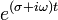2.10d
141   -4, -8   In Example 5.4, there is a sign error in one of the terms 2.10c
145   8-9   At end of Example 5.5, eigenvalue approximations are incorrect 2.10d
145   8-9   At end of Example 5.5, real part of eigenvalues has incorrect sign 2.10e
147   10-15   Impulse response does not include the direct term in the proper manner 2.11b
148   2   In the caption for Figure 5.7, "drive" should be "driven" 2.10c
157   7   In equation (5.26), the u(k) term in the integral should be u(tau) 2.10c
158   13, 22   In Example 5.11, the parameter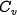should be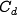2.11b
158   -8   After equation (5.31), rolling friction term disappears if v = 0 2.10c
158   -7   After equation (5.31), the equilibrium velocity should bem/s 2.11b
158   -5   After equation (5.31), the parameterlisted in the text should be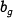2.11b
161   3   In Example 5.12, expression non-equilibrium condition has wrong symbols 2.10d
162   7   In equation (5.37), the parametershould be2.11b
163   10   In equation (5.39), Coriolis term should be + C(q, \dot q) 2.10d
165   -4   In Exercise 5.8d, the left hand side of the first equation should be x(k+1) 2.10c
178   -12   In Example 6.4, the initial control signal is u(0) = k_r r = \omega_c^2 r 2.11b
179   -12   In equation (6.17), elements of the output matrix should be in the opposite order 2.10d
179   -8   In displayed equation after equation (6.17), a_l should be a_1 2.10d
182   16-20, 25   In Example 6.5, reference variable should be L_d instead of r 2.10d
182   3   In Example 6.5, definitions of z1 and z2 are switched 2.11a
182   17   In second line of displayed equation, missing minus sign in front of the gain matrix 2.10d
182   21   In the last paragraph of Example 6.5, r_h should be u 2.10d
183   4   In the caption for Figure 6.7, L_e=20 should be L_e=30 2.10d
183   -7   In equation (6.22),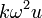should be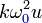2.11b
184   17   For underdamped second order system, eigenvalues are listed incorrectly 2.10d
185   -3   The formula given for the rise time of a second order system in Table 6.1 is only approximate 2.11a
186   5   Before Example 6.6, "is Q-value" should be "its Q-value" 2.10d
186   -3   In Example 6.6, the output equation has 'x' for the state instead of 'c' 2.10c
188   -2   In Example 6.7, the A matrix has an error in the (3,4) entry 2.11b
189   5   In Example 6.7, the open loop eigenvalues are incorrect 2.11b
189   15   In Example 6.7, the imaginary part of the first pair of eigenvalues is missing factor of i 2.10d
191   -5   In Example 6.8, the state space dynamics have errors in the trigonometric terms 2.10c
192   4-14   In Example 6.8, the state error should be \xi and the input should be F 2.10d
192   -15   Diagonal entries for Q_v should be rho 2.10c
196   19, 23   In Example 6.10, the system input for the linearized model should be w instead of u 2.11a
196   -1   In Example 6.10, the reference gain should be k_r = a_1/b 2.11b
204   6   In Example 7.1, the output equation has 'x' for the state instead of 'c' 2.10c
206   2   In expression for W_0, (3, 1) entry should be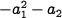(remove factor ofin second term) 2.10c
207   13   "going zero" → "going to zero" 2.10d
213   8   Estimator dynamics in Theorem 7.3 are missing B k_r r term 2.10c
217   -6, -5   In Example 7.5, descriptions for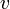(disturbance) andare switched 2.10c
219   -5   Change gain matrix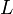tofor consistency 2.10d
220   17   In expression for B(t), missing parenthesis at end of subscripted expression 2.11a
220   -12   Above displayed equation for the gain scheduled control law,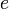should be replaced by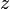2.10d
221   -15   In Example 7.5, "where were" → "which were" 2.10d
224   4   Output in Kalman decomposition for Example 7.7 is missing state error term 2.10c
225   -4   "phenomena knows" → "phenomena known" 2.10c
229   10   Henrik Bode should be Hendrik Bode 2.11a
233   -8   In equation (8.6), matrix inverse is computed incorrectly 2.10d
235   14   In displayed equation above equation (8.9), exponential term on right hand side should be(no minus sign) 2.10d
236   -9   In Example 8.2, resister should be resistor 2.11b
238   -9   In equation (8.15), second order partial derivative is written incorrectly 2.10c
238   -1   In Example 8.4, boundary condition and coefficients for psi(x) are incorrect 2.10c
241   -12   Before Example 8.5, "related" → "relate" 2.10d
242   7   In Example 8.5, leading term in denominator for H_\theta F should be cubic in s 2.10d
247   2   In Example 8.6, numerator gain term for G_ur should be k_r instead of k_1 2.10d
247   11   Last equation in Example 8.6 has sign error in the second term 2.10c
247   11   Last equation in Example 8.6 has errors in numerator expressions 2.10d
249   6   In Example 8.7, remove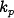from numerator of expression for2.10d
256   -10,-9   In Example 8.9, u should be v in the first displayed equation and the following line 2.11a
262   -2   In Exercise 8.2, text should read "transfer function fromto" (instead of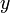) 2.10d
263   -13   In Exercise 8.5, k in the denominator of G(s) should be (k+1) 2.10c
264   9   In Exercise 8.6, second term in expression for b_n should be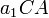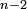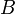2.10d
272   10, 11   In the paragraph above Example 9.3, G(*) should be L(*) 2.11a
272   -1   In Example 9.3, intersection of negative real axis should be at-0.5. 2.10d
273   -5   In Example 9.4, "that that" → "that" 2.10d
274   -2   In right hand expression of last displayed equation of Example 9.4,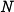in the denominator should be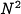2.10d
275   1   In the caption for Figure 9.7, the transfer function should match equation (9.4) 2.11a
275   3   In caption for Figure 9.7, "intersections" → "intersects" 2.10d
275   -5   In Example 9.5, "it is seems" → "it seems 2.10d
282   -11, -9   In Example 9.9, 'gain margin' g_m should be 'stability margin' s_m 2.10d
282   -7   In Example 9.9, the gain margin for Figure 9.12 should be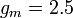2.10d
283   12   In equation (9.8), the right hand side should be evaluated at omega0 2.11a
283   -6   Missing parenthesis in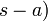2.10d
284   -13   In Example 9.10, transfer function zero is at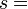2.10d
289   7   In equation (9.14),should be2.10d
292*   10   In Exercise 9.7,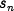should be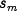2.11a
296   1   Caption for Figure 10.3b should be "Derivative action" 2.10c
297   11   After equation (10.5), low frequency limit bound be 1/T_d instead of T_d 2.10c
299   -10   Closed loop time constant after equation (10.6) has an extra factor of 2 2.11a
300   4   The b k_i term in the characteristic polynomial has an extra factor of s 2.10c
300   7   In displayed equation above equation (10.7), the final term should be(not squared) 2.10d
302   0   In Figure 10.7b,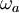should be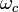2.10c
304   -8   In Example 10.4, z should be zeta 2.11a
304   -1   In Example 10.4, proportional gain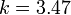should be2.10d
305   -1   In equation (10.12),should be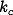2.11a
306   1   Right after equation (10.12),should be2.11a
308   -9   In Section 10.5, PID derivative filter should have time constant2.10d
309   9   In equation (10.4), upper limit of the integral should be t, not infinity 2.11a
310   -4   The expressions for the PID parameters for the op amp implementation are incorrect 2.10d
318   -4 to -1   Reference input not included in Figure 11.2 and dimensions of blocks are listed incorrectly 2.10c
319   0   In Figure 11.3, the label 'y' at the input to P_2 should be removed 2.10c
326   -1   In equation (11.11), delete extraneous "[rad]" 2.10d
328   -11   In Example 11.5, "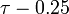" should be "" 2.10c
328   -6   In Example 11.5, "dotted" line is actually "dash-dotted" line 2.11a
329   3,5   In the Figure 11.10 caption and Example 11.5 text, "dotted" line is actually "dash-dotted" line 2.11a
330   2   In the caption for Figure 11.11, Fz should be F1 2.10c
334   0   In Figure 11.13a, curves should be labeled with z instead of b 2.10d
338   -6   At end of Example 11.11, "the can be achieved" should be "that can be achieved" 2.10d
339   -9   In expression for I_1, limits have an extra factor of i 2.10c
340   3-9   Contour integral in derivation of Bode's integral formula is analyzed incorrectly 2.10c
341   -8   In Example 11.12, value for -mg should be -39.2 2.11a
341   -5   In Example 11.12, transfer functions for inner loop and outer loop dynamics are incorrect 2.11b
342   5   In Example 11.12, error should be in, not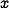2.11a
342   13   In Example 11.12, after displayed equation for C_o(s), frequency where phase lead flattens out should be b_o/10 2.10d
342   -16   In Example 11.12, transfer functions for inner loop and outer loop dynamics are incorrect 2.11b
342   -10   In Example 11.12, gain term inshould be -2 instead of 0.8 2.11a
344   -4   In Exercise 11.5, final expression for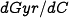should be2.11a
345   -10   In Exercise 11.10, controller gain at frequencyshould be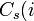2.10d
357   -6, -8   For Youla parameterization, P = b(s)/a(s) = B(s)/A(s) and C0 = G0(s)/F0(s) 2.10c
359   -6, -4   In equation (12.13) and the displayed equation above it, T should be -T 2.10d
361   13   Missing end parenthesis at end of sentence 2.10c
363   17   In Example 12.8, repeated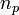in denominator of transfer function forshould be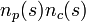2.10d
365   -13   The reference to the maximum complementary sensitivity should be to equation (12.7) 2.10c
366   -16   In equation (12.16), a is not defined and a factor of a is missing in numerator the last term 2.10c
367   -8   In equation (12.18), a factor of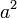is missing from the numerator 2.10c
368   7   In equation (12.21), final term in expression for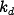should be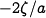2.10d
369   -12   Replace "dashed" with "shaded" in the description of Figure 12.16a 2.10c
371   -4   Reference to Figure 12.17b should be to Figure 12.17 (right) 2.10c
376   2   In Exercise 12.11, first term in numerator forshould be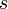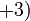2.10d

### Second printing

Page Line Erratum Version
21   7   Stanford one the competition should be Stanford won the competition 2.10c
29   -12   Missing space after sentence ending with "controlled differential equation" 2.10b
52   0   In Figure 2.16, theta should be O 2.10b
85   -7   Equation (3.24) should have c in the numerator instead of c0 2.10b
87   3   In equation (3.27), the state used for the output should be c, not x 2.10b
97   -11, -4   Equation (4.4) is labeled incorrectly 2.10b
107   12-15   In Example 4.6, u_d is not computed correctly 2.10b
115   -5   In Example 4.11, Taylor series term is missing factor of 1/2 2.10b
118   17   After equation (4.18), solution trajectory should be x(t; a) instead of x(t: a) 2.10b
121   5 to 6   Global Lyapunov stability requires additional conditions 2.10b
152   -5   In the complex input u = exp(s t), s should be i omega, not i omega t 2.10b
157   -15   Missing factor of B in displayed equation after equation (5.28) 2.10b
171   8   In equation (6.5), the 4, 3 entry of W_r should be g m^2 l^2 M_t/mu 2.10b
174   -3   In Example 6.3, z_2 should be x_1/omega instead of x_2/omega 2.10b
179   -3   Reference to equation (6.17) should be to equation (6.15) 2.10b
216   -7 to -3   F R_v F^T term dropped in proof of Theorem 7.4 2.10b
221   -12, -6   In Example 7.6, the coefficient in front of thetadot should be v/b 2.10b
222   4, 5   In equation (7.25), the last two endpoint equations are not correct 2.10b
228   13   In Exercise 7.11, output to check is the feedforward signal 2.10b
228   -12   In Exercise 7.13, missing equals sign in covariance specification 2.10b
234   -12   In displayed equation above equation (8.7), U should be u in the expression for the output 2.10b
237   4   In Figure 8.3, the gain falls off at omega = a R1 k / R2 2.10b
237   5   In Example 8.3, a = 10 rad/s 2.10b
238   8   In Example 8.3, a = 10 rad/s 2.10b
240   -12   After equation (8.17), "looses" should be "loses" 2.10b
248   2   Formula for Ger should have the ' on dp, not dc 2.10b
248   7   Formula for Ged should not have the ' on dc and is missing a minus sign 2.10b
254   -7   Replace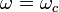with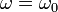2.10b
255   8   Replacewith2.10b
256   0, 5, 6   In Figure 8.15 and accompanying text a should be omega0 2.10b
264   16   In Exercise 8.8, second transfer function should be G_yn instead of G_yd 2.10b
276   -6, -2   In Example 9.6, the critical gain is k = 0.5 instead of k = 1 2.10b
277   4   In Figure 9.8, the gain of the system is k = 1 2.10b
278   2   In proof of Theorem 9.3, "residues of the poles of is" should be "residue for the poles is" 2.10b
284   -10   In Example 9.10, there is an extra factor of a in the second term of the step response 2.10b
335   11, 13   In Example 11.9, the bound on the gain crossover frequency should be 6.48 rad/sec 2.10b
351   8   Riemann sphere has radius (not diameter) 1 2.10b
351   10   d(P_1, P_2) is the longest chordal distance, not shortest 2.10b
357   0   In Figure 12.8, the signs of A and B are reversed 2.10b
363   -18   In Example 12.8, process zero is at s = -2 2.10b
363   -17   In Example 12.8, controller zero is at s = 3.5 2.10b
364   -10 to -4   In Example 12.9, poles are at -a, -p1, -p2 2.10b
367   -1   Remove extra word "in" 2.10b
371   -11   After equation (12.23), generalized error is z, not w 2.10b
379   23   Desborough is misspelt (p 380, line 1 in online version) 2.10b

### First printing

Page Line Erratum Version
15   6   In Figure 1.11, caption refers to "numbers in circles" that are not present in figure 2.9d
19   -11   The Wright brothers made their first successful flight in 1903, not 1905 2.9d
25   -1   In Exercise 1.1, "loosing" should be "losing" 2.9d
32   12   In discussion on disturbance signals, "can" should be "cannot" 2.9d
62   -6   In Exercises 2.4 and 5.9, the (2,1) entry of the dynamics matrix should be ab-b 2.9d
63   -8   In Exercise 2.7, reference to inverted pendulum may be confusing 2.9d
80   5   In Equation (3.19), the second term in the window dynamics should contain \rho c 2.9d
91   -6   In Exercise 3.2: use observable form, delta is the steering angle and "title" should be "tilt" 2.9d
92   -9   State space dynamics in Exercise 3.5 are not correct 2.9d
106   7   Solution for x_2j in block diagonal form discussion has sign errors 2.9d
107   12-23   Closed loop dynamics matrix is incorrect in Example 4.6 2.9d
108   8   Missing γ in equation (4.9) 2.9d
110   11, 13   In Example 4.8, the linearized dynamics should contain 3 r_e, not 2 r_e 2.9d
112   -1   In Example 4.9, V(x) should be V(z) 2.9d
117   3   Typo in the caption for Figure 4.15 2.9d
126   -5   In Exercise 4.1, x_0 should not be subtracted from time-shifted solution 2.9d
126   -5   In Exercise 4.1, tau should be defined as t-t_0 2.9d
127   5   In Exercise 4.2, a_e should be a 2.9d
127   15   Sign error in second Lyapunov function in Exercise 4.4 2.9d
128   3   In Exercise 4.6, Pm/J should be taken as 1 and factor of 1/2 is misplaced 2.9d
129   -2   In Exercise 4.14c, the transformation T and its inverse are swapped 2.9d
131   -15   Extraneous text "!linear" in Section 5.1 2.9d
135   12   In equation (5.5), the upper limit of the integral should be t 2.9d
144   -7   Example 5.5 includes a damping term not shown in Figure 5.4 2.9d
144   -4   In Example 5.5 and 5.6, m1 and m2 should be m 2.10a
145   5   In Examples 5.5 and 5.6, dynamics in modal form should use z, not x 2.9d
146   -15   Equation (5.15) is linear in the initial conditions and *input* 2.10a
148   -1   In Example 5.5 and 5.6, m1 and m2 should be m 2.10a
149   8   In Examples 5.5 and 5.6, dynamics in modal form should use z, not x 2.9d
154   -10   In Example 5.8, the third nodal equation should be deleted 2.10a
155   0   The labels for v2 and v3 are misplaced in Figure 5.12 2.10a
156   -10   In AFM dynamics, c and k are missing subscript 2 2.9d
157   7   Missing factor of A in second term of equation (5.26) 2.10a
164   -11   Parameter values are missing in Exercise 5.3 2.9d
164   -3   Remove part (a) in Exercise 5.6 2.9d
165   11   In Exercise 5.7, the 5% settling time should be 3 tau instead of 2 tau 2.9d
165   16   In Exercise 5.8, the initial condition is x_0 2.9d
166   3   In Exercises 2.4 and 5.9, the (2,1) entry of the dynamics matrix should be ab-b 2.9d
168   12   The control matrix in the equilibrium set should be 'B' instead of 'b' 2.10a
169   -12   In heuristic derivation of reachability test, α is missing subscript 2.9d
180   -2   Formula for k_r in equation (6.21) of Theorem 6.3 is incorrect 2.9d
181   9   Missing 's' in expression for characteristic polynomial in discussion of eigenvalue assignment 2.9d
183   -7   In equation (6.22), k omega^2 should be k omega_0^2 2.9d
184   -10   In equation (6.24), the sign of the sin(omega t) term is incorrect for zeta less than one 2.9d
185   4   Values of zeta in the caption for Figure 6.8 don't match the figure 2.9d
188   -2   Dynamics matrix in Example 6.7 has errors in (3,4) and (4,4) entries 2.9d
189   22   Numerical gains for Example 6.7 are incorrect 2.9d
197   3   In Figure 6.15, reference velocity is v_r = 20 m/s 2.10a
198   14   In Exercise 6.4, need to assume A is invertible and C A^-1 B is nonzero 2.9d
199   -1, -2   In AFM dynamics, c and k are missing subscript 2 2.9d
200   9   In Exercise 6.13, R should be removed and rho should be rho_1 2.9d
206   10,11   In the last paragraph of Section 7.1, "reachable" should be replaced by "observable" 2.9d
209   2   In Example 7.2, the observer gain is given by equation (7.11) 2.9d
214   -13   In Example 7.4, hats are missing in displayed equation for u 2.10a
220   12   In computation of error dynamics, u_ff argument is missing 2.9d
220   -13   Minus sign is missing in gain scheduled feedback 2.9d
224   -7   References to first and second equations are switched in computer implementation discussion 2.9d
240   -16, -13   Sign error in equation (8.17) - (sI - A) should be (A - sI) 2.9d
240   -11   Explanation of the lack of zeros when B or C is full rank is confusing 2.9d
241   -4, -6   In Example 8.5, q should be replaced by theta 2.9d
247   -1   In section on pole/zero cancellations, pole and zero are at s = -a 2.10a
248   1   In section on pole/zero cancellations, pole and zero are at s = -a 2.10a
249   3   Location of the process pole is missing a zero in figure caption for cruise control example 2.9d
250   -16   Missing parenthesis in exponential for output signal 2.10a
253   -1   In description of Bode plot for second order transfer function, 'a' should be omega_0 2.10a
254   12   In description of Bode plot for second order transfer function, 'a' should be omega_0 2.10a
254-5   -10 to 9   In Example 8.8, explanation of effects of poles and zeros is incorrect and confusing 2.10a
256   1   Caption for Figure 8.15b is missing an s in the numerator 2.9d
257   5   In Example 8.9, the expression for sigma is not correct 2.10a
272   8   Before Example 9.3, range of theta should be -pi/2 to pi/2 2.10a
277   13   In Theorem 9.3, N should be Z 2.9d
278   3   Missing factor of 2 pi in residue formula for proof of Theorem 9.3 2.10a
288   -4, -3   Exponential signal is incorrect on the left-hand side of harmonic expansion equation 2.9d
292   18   In Exercise 9.9, 10/a should be 1/tau 2.9d
307   -9   Toward end of Section 10.4, k_i should be k_t 2.9d
308   0   Figure 10.11 is missing filter on derivative term 2.9d
310   -4   In Figure 10.13, the indices on the resistors and capacitors are incorrect 2.9d
311   -3   Missing ydot after equation (10.16) 2.9d
313   8,9   In Exercise 10.1, the second term in the denominator should be kp not kd 2.9d
314   -5   In Exercise 10.11, the dynamics for x_2 should test for e less than e_0, not e less than 1 2.9d
315   -8   Extraneous 'LDH' and comment in the text 2.9d
319   0   Arrow labeled u_fd in Figure 11.3 is in the wrong direction 2.9d
319   4, -2   The signal u_ff should be u_fr in the caption for Figure 11.3 and associated text 2.9d
319   -7   Extraneous text "!design" in Chapter 12 2.9d
323   -9   Maximum sensitivity occurs at frequency omega_ms not omega_sc 2.9d
325   -9   Proportional gain in Example 11.4 transfer function should be kp 2.9d
330   5   Denominator of vectored thrust process model should be Js^2 2.9d
336   -8   Extraneous text "!design" in Chapter 12 2.9d
345   12   Exercise 11.4 should be Example 11.4 in Exercise 11.9 2.9d
345   14   T_d should be T_f in Exercise 11.9 2.9d
345   -5   Numerator and denominator are switched in Exercise 11.11 2.9d
351   2,3   Caption for Figure 12.4 doesn't quite match figure 2.9d
356   6, 12   In Example 12.6, n = -1 should be n = 1 2.9d
363   -11   "Assigning a closed loop zero" should be "assigning a closed loop pole" 2.9d
369   -1   Extraneous text "!design" in Chapter 12 2.9d
378   15,16   Bennett's books on the history of control were published in 1979 and 1993 2.10a